Estimating sum and difference

Chapter 1 Class 6 Knowing our Numbers
Concept wise

General rule says

• For a number in tens, we round off to tens.
• For a number in hundred, we round off to hundred.
• For a number in thousands, we round off to thousands.

## 479 × 81

According to general rule,

• 479 is rounded off to nearest hundred

∴ 479 → 500

• 81 is rounded off to nearest ten

∴ 81 → 80

So, Estimated Product = 500 × 80

= 5 × 100 × 8 × 10

= (5 × 8) × 1000

= 40 × 1000

= 40000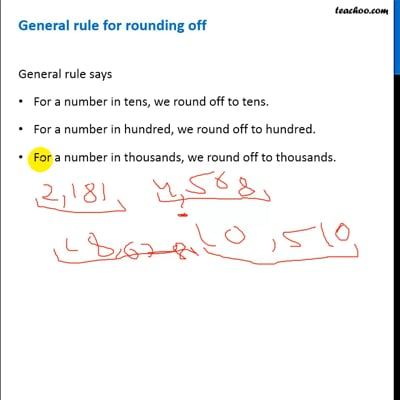This video is only available for Teachoo black users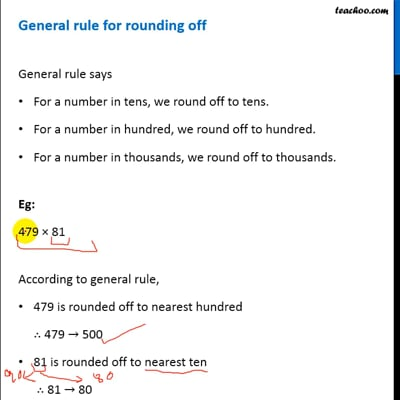This video is only available for Teachoo black users

Let's look at some more examples

## 37 × 1889

According to general rule,

• 37 is rounded off to nearest ten

∴ 37 → 40

• 1889 is rounded off to nearest thousand

∴ 1889 → 2000

So, Estimated Product = 40 × 2000

= 4 × 10 × 2 × 1000

= (4 × 2) × 10000

= 8 × 10000

= 80000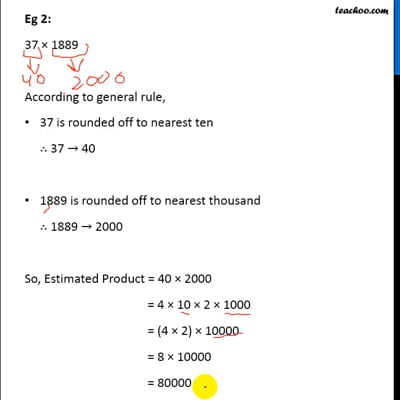This video is only available for Teachoo black users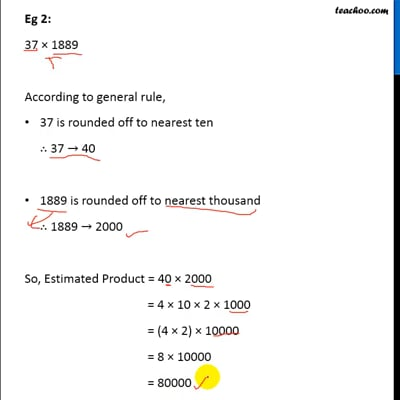This video is only available for Teachoo black users

## 232 + 4821

According to general rule,

• 232 is rounded off to nearest hundred
∴ 232 → 200
• 4821 is rounded off to nearest thousand
∴ 4821 → 5000

So, Estimated Sum = 200 + 5000

= 5200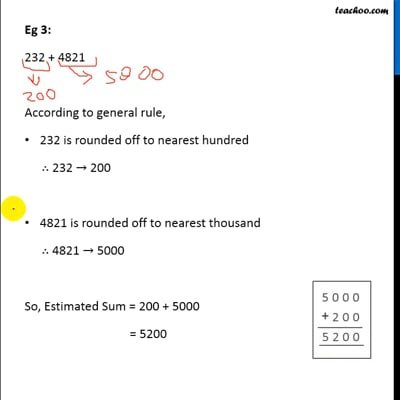This video is only available for Teachoo black users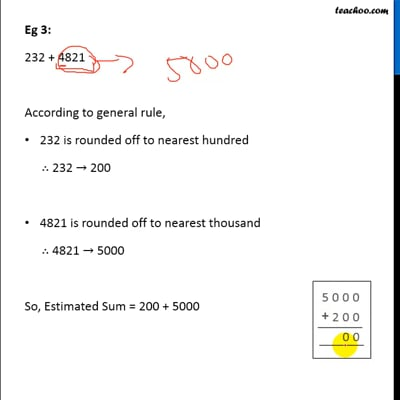This video is only available for Teachoo black users

## 1089 + 6871

According to general rule,

• 1089 is rounded off to nearest thousand,
∴ 1089 → 1000
• 6871 is rounded off to nearest thousand
∴ 6871 → 7000

∴ Estimated Sum   = 1000 + 7000

= 8000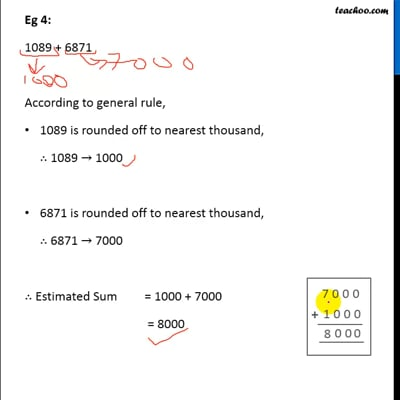This video is only available for Teachoo black users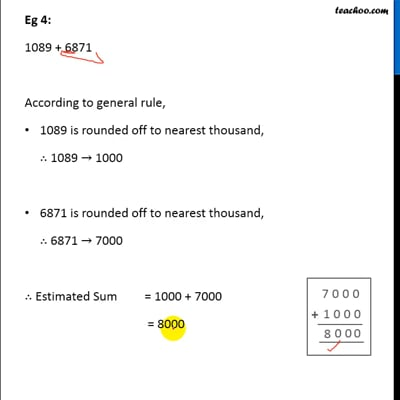This video is only available for Teachoo black users

Eg 5:

9560 − 421

According to general rule,

• 9560 is rounded off to nearest thousand,
∴ 9560 → 10,000
• 421 is rounded off to nearest hundred,
∴ 421 → 400

∴ Estimated Difference = 10,000 – 400

= 9600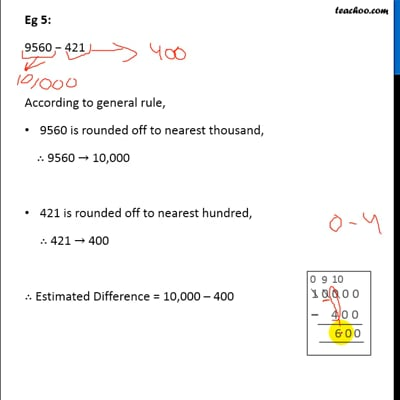This video is only available for Teachoo black users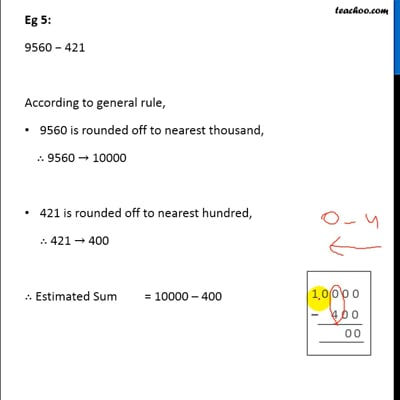This video is only available for Teachoo black users

### Transcript

General rule for rounding off General rule says For a number in tens, we round off to tens. For a number in hundred, we round off to hundred. For a number in thousands, we round off to thousands. Eg: 479 × 81 According to general rule, 479 is rounded off to nearest hundred ∴ 479 → 500 81 is rounded off to nearest ten ∴ 81 → 80 So, Estimated Product = 500 × 80 = 5 × 100 × 8 × 10 = (5 × 8) × 1000 = 40 × 1000 = 40000 Eg 2: 37 × 1889 According to general rule, 37 is rounded off to nearest ten ∴ 37 → 40 1889 is rounded off to nearest thousand ∴ 1889 → 2000 So, Estimated Product = 40 × 2000 = 4 × 10 × 2 × 1000 = (4 × 2) × 10000 = 8 × 10000 = 80000 Eg 3: 232 + 4821 According to general rule, 232 is rounded off to nearest hundred ∴ 232 → 200 4821 is rounded off to nearest thousand ∴ 4821 → 5000 So, Estimated Sum = 200 + 5000 = 5200 Eg 4: 1089 + 6871 According to general rule, 1089 is rounded off to nearest thousand, ∴ 1089 → 1000 6871 is rounded off to nearest thousand, ∴ 6871 → 7000 ∴ Estimated Sum = 1000 + 7000 = 8000 Eg 5: 9560 − 421 According to general rule, 9560 is rounded off to nearest thousand, ∴ 9560 → 10,000 421 is rounded off to nearest hundred, ∴ 421 → 400 ∴ Estimated Difference = 10,000 – 400 = 9600